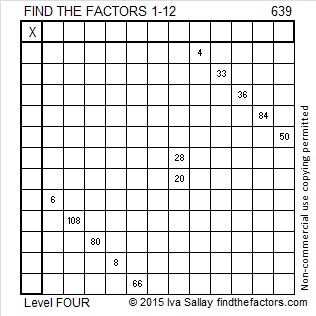# 639 and Level 4

639 is the sum of the 20 prime numbers from 2 to 71, its largest prime factor.

639 is made from 3 numbers that are divisible by 3 so 639 is divisible by 3 AND by 9.

Puzzle #639 has a tricky clue in it, but I’m sure you can still solve it.Print the puzzles or type the solution on this excel file: 12 Factors 2015-10-05

—————————————————————————————————

• 639 is a composite number.
• Prime factorization: 639 = 3 x 3 x 71, which can be written 639 = (3^2) x 71
• The exponents in the prime factorization are 2 and 1. Adding one to each and multiplying we get (2 + 1)(1 + 1) = 3 x 2  = 6. Therefore 639 has exactly 6 factors.
• Factors of 639: 1, 3, 9, 71, 213, 639
• Factor pairs: 639 = 1 x 639, 3 x 213, or 9 x 71
• Taking the factor pair with the largest square number factor, we get √639 = (√9)(√71) = 3√71 ≈ 25.278449.—————————————————————————————————This site uses Akismet to reduce spam. Learn how your comment data is processed.